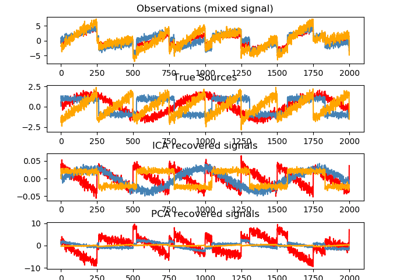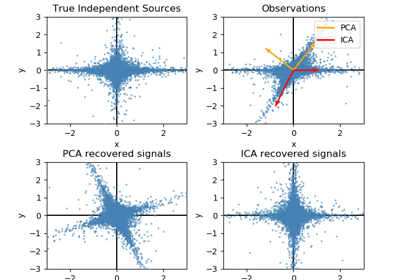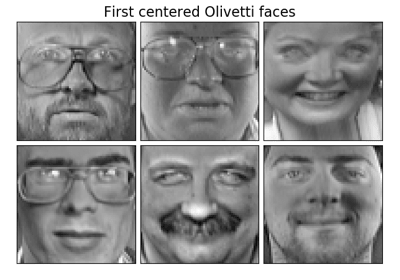/scikit-learn

# sklearn.decomposition.FastICA

`class sklearn.decomposition.FastICA(n_components=None, algorithm=’parallel’, whiten=True, fun=’logcosh’, fun_args=None, max_iter=200, tol=0.0001, w_init=None, random_state=None)` [source]

FastICA: a fast algorithm for Independent Component Analysis.

Read more in the User Guide.

Parameters: `n_components : int, optional` Number of components to use. If none is passed, all are used. `algorithm : {‘parallel’, ‘deflation’}` Apply parallel or deflational algorithm for FastICA. `whiten : boolean, optional` If whiten is false, the data is already considered to be whitened, and no whitening is performed. `fun : string or function, optional. Default: ‘logcosh’` The functional form of the G function used in the approximation to neg-entropy. Could be either ‘logcosh’, ‘exp’, or ‘cube’. You can also provide your own function. It should return a tuple containing the value of the function, and of its derivative, in the point. Example: def my_g(x): return x ** 3, 3 * x ** 2 `fun_args : dictionary, optional` Arguments to send to the functional form. If empty and if fun=’logcosh’, fun_args will take value {‘alpha’ : 1.0}. `max_iter : int, optional` Maximum number of iterations during fit. `tol : float, optional` Tolerance on update at each iteration. `w_init : None of an (n_components, n_components) ndarray` The mixing matrix to be used to initialize the algorithm. `random_state : int, RandomState instance or None, optional (default=None)` If int, random_state is the seed used by the random number generator; If RandomState instance, random_state is the random number generator; If None, the random number generator is the RandomState instance used by `np.random`. `components_ : 2D array, shape (n_components, n_features)` The unmixing matrix. `mixing_ : array, shape (n_features, n_components)` The mixing matrix. `n_iter_ : int` If the algorithm is “deflation”, n_iter is the maximum number of iterations run across all components. Else they are just the number of iterations taken to converge.

#### Notes

Implementation based on `A. Hyvarinen and E. Oja, Independent Component Analysis: Algorithms and Applications, Neural Networks, 13(4-5), 2000, pp. 411-430`

#### Examples

```>>> from sklearn.datasets import load_digits
>>> from sklearn.decomposition import FastICA
>>> transformer = FastICA(n_components=7,
...         random_state=0)
>>> X_transformed = transformer.fit_transform(X)
>>> X_transformed.shape
(1797, 7)
```

#### Methods

 `fit`(X[, y]) Fit the model to X. `fit_transform`(X[, y]) Fit the model and recover the sources from X. `get_params`([deep]) Get parameters for this estimator. `inverse_transform`(X[, copy]) Transform the sources back to the mixed data (apply mixing matrix). `set_params`(**params) Set the parameters of this estimator. `transform`(X[, y, copy]) Recover the sources from X (apply the unmixing matrix).
`__init__(n_components=None, algorithm=’parallel’, whiten=True, fun=’logcosh’, fun_args=None, max_iter=200, tol=0.0001, w_init=None, random_state=None)` [source]
`fit(X, y=None)` [source]

Fit the model to X.

Parameters: `X : array-like, shape (n_samples, n_features)` Training data, where n_samples is the number of samples and n_features is the number of features. `y : Ignored` self
`fit_transform(X, y=None)` [source]

Fit the model and recover the sources from X.

Parameters: `X : array-like, shape (n_samples, n_features)` Training data, where n_samples is the number of samples and n_features is the number of features. `y : Ignored` `X_new : array-like, shape (n_samples, n_components)`
`get_params(deep=True)` [source]

Get parameters for this estimator.

Parameters: `deep : boolean, optional` If True, will return the parameters for this estimator and contained subobjects that are estimators. `params : mapping of string to any` Parameter names mapped to their values.
`inverse_transform(X, copy=True)` [source]

Transform the sources back to the mixed data (apply mixing matrix).

Parameters: `X : array-like, shape (n_samples, n_components)` Sources, where n_samples is the number of samples and n_components is the number of components. `copy : bool (optional)` If False, data passed to fit are overwritten. Defaults to True. `X_new : array-like, shape (n_samples, n_features)`
`set_params(**params)` [source]

Set the parameters of this estimator.

The method works on simple estimators as well as on nested objects (such as pipelines). The latter have parameters of the form `<component>__<parameter>` so that it’s possible to update each component of a nested object.

Returns: self
`transform(X, y=’deprecated’, copy=True)` [source]

Recover the sources from X (apply the unmixing matrix).

Parameters: `X : array-like, shape (n_samples, n_features)` Data to transform, where n_samples is the number of samples and n_features is the number of features. `y : (ignored)` Deprecated since version 0.19: This parameter will be removed in 0.21. `copy : bool (optional)` If False, data passed to fit are overwritten. Defaults to True. `X_new : array-like, shape (n_samples, n_components)`

## Examples using `sklearn.decomposition.FastICA`Blind source separation using FastICAFastICA on 2D point cloudsFaces dataset decompositions